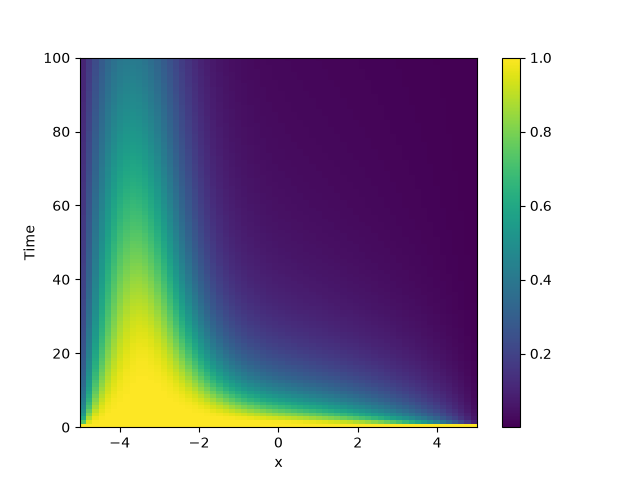# 2.16. Diffusion equation with spatial dependence

This example solve the Diffusion equation with a heterogeneous diffusivity:

$\partial_t c = \nabla\bigr( D(\boldsymbol r) \nabla c \bigr)$

using the PDE class. In particular, we consider $$D(x) = 1.01 + \tanh(x)$$, which gives a low diffusivity on the left side of the domain.

Note that the naive implementation, PDE({"c": "divergence((1.01 + tanh(x)) * gradient(c))"}), has numerical instabilities. This is because two finite difference approximations are nested. To arrive at a more stable numerical scheme, it is advisable to expand the divergence,

$\partial_t c = D \nabla^2 c + \nabla D . \nabla c$from pde import PDE, CartesianGrid, MemoryStorage, ScalarField, plot_kymograph

# Expanded definition of the PDE
diffusivity = "1.01 + tanh(x)"
term_1 = f"({diffusivity}) * laplace(c)"